# JP2006317418A - Image measuring device, image measurement method, measurement processing program, and recording medium - Google Patents

Image measuring device, image measurement method, measurement processing program, and recording medium Download PDF

## Info

Publication number
JP2006317418A
JP2006317418A JP2005143317A JP2005143317A JP2006317418A JP 2006317418 A JP2006317418 A JP 2006317418A JP 2005143317 A JP2005143317 A JP 2005143317A JP 2005143317 A JP2005143317 A JP 2005143317A JP 2006317418 A JP2006317418 A JP 2006317418A
Authority
JP
Japan
Prior art keywords
image
measured
straight line
measurement
means
Prior art date
Legal status (The legal status is an assumption and is not a legal conclusion. Google has not performed a legal analysis and makes no representation as to the accuracy of the status listed.)
Pending
Application number
JP2005143317A
Other languages
Japanese (ja)
Inventor
Yukinobu Ishino

Original Assignee
Nikon Corp

Priority date (The priority date is an assumption and is not a legal conclusion. Google has not performed a legal analysis and makes no representation as to the accuracy of the date listed.)
Filing date
Publication date
Application filed by Nikon Corp, 株式会社ニコン filed Critical Nikon Corp
Priority to JP2005143317A priority Critical patent/JP2006317418A/en
Publication of JP2006317418A publication Critical patent/JP2006317418A/en
Application status is Pending legal-status Critical

• 238000000691 measurement method Methods 0 title 1
• 239000002609 media Substances 0 title 1
• 238000003384 imaging method Methods 0 abstract 4
• 238000009740 moulding (composite fabrication) Methods 0 abstract 1

## Images

•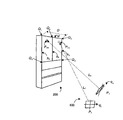•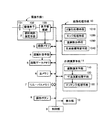•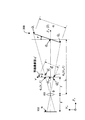•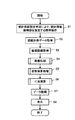•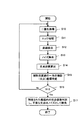•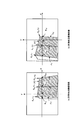•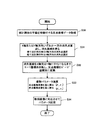•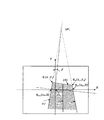•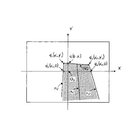•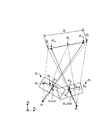•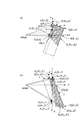•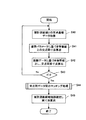•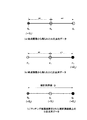••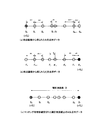## Abstract

Dimension measurement is possible only with qualitative shape information of a measured object.
An object to be measured 200, a part to be measured designating unit 2 for designating a straight line portion of the object to be measured, an image capturing unit 1 for capturing an image of the object to be measured, the image capturing unit, and an object on the object to be measured The distance measuring means 4 for measuring the distance to the point to be measured, the straight line detecting means 1030 for detecting a straight line component based on the captured image, the straight line detected by the straight line detecting means, and the measured portion specifying means Based on the intersection coordinates specified by the image processing means and the image processing means having the intersection coordinate specifying means for specifying the intersection coordinates with the specified straight line portion, a plane characterizing the object to be measured is formed, Based on the posture calculation means 110 for calculating the posture parameter of the plane with respect to the imaging surface, the posture parameter calculated by the posture calculation means and the distance detected by the distance measurement means, And a dimension calculating means 120 for calculating the dimensions of the measured linear.
[Selection] Figure 1

## Description

The present invention relates to an image measuring apparatus, an image measuring method, and a recording medium on which a measurement processing program is recorded, which measures the size of an object / shape based on a captured image.

Conventionally, as a method for measuring an image of a three-dimensional object shape, various range finder measurement methods and stereo photo measurement methods are known. Stereo image measurement includes, for example, Patent Document 1 in which the same subject is imaged from two different viewpoints, and the position of the measurement point is measured based on the principle of triangulation using the parallax between the viewpoints.

In recent years, digital cameras have become widespread and are easily used in the surveying field. Patent Documents 2, 3, and 4 are examples of measuring the size of an object shape based on an image captured by a digital camera. Patent Document 2 discloses an oblique distance between measurement points in a configuration including a distance measuring unit that irradiates a subject with pulse-modulated laser light, receives the reflected light, and measures a distance, an azimuth sensor, an inclination sensor, and the like. A distance measuring device such as a horizontal angle that a normal surveying device has is proposed. Patent Document 3 proposes that a reference rectangular body whose dimensions are known in advance is imaged together with the object to be measured, the captured image is taken into a personal computer, and a part to be measured is input and specified to obtain an actual dimension. . Patent Document 4 is an example of a digital camera that can measure an object size using an autofocus function. This is a proposal for obtaining a dimension between objects based on an image picked up so that two edges in a subject to be measured are included in a focus area.

Further, Patent Document 5 is a proposal for calculating a posture parameter of an imaging surface with respect to a rectangular plane with a monocular camera and detecting a coordinate position of an indicated point on the rectangular plane. The three posture parameters in the real space can be calculated from the four right-angled points that characterize the rectangular shape.
Japanese Patent No. 2004-170277 Japanese Patent No. 2001-124544 Japanese Patent No. 2004-212142 JP-A-9-133516 Japanese Patent No. 2001-148025

However, the conventional example has the following problems.
In the stereo photo measurement method, when determining the left and right gaze directions, it is very difficult to determine where the points in the right image correspond to in the left image, so-called corresponding point determination (matching processing). There is a problem that ambiguity remains in the determination of corresponding points. In addition, complicated calculation processing must be performed, resulting in a problem that the apparatus becomes large and the cost is high.

According to Patent Document 1, it is necessary to set an evaluation function of distance data by laser ranging to find the difficulty in assigning corresponding points in stereo photography, and even if three-dimensional measurement can be realized precisely, the apparatus becomes large and complicated. It is necessary to perform an operation.

Patent Document 2 has a drawback that the cost is increased because it is necessary to provide an attitude sensor in the imaging means. Furthermore, since the measurement value is a method of calculating dimensions by indicating two measurement points on the image displayed on the monitor, there arises a problem that the operation of indicating is troublesome with a small monitor.

In Patent Document 3, it is necessary to image a reference body with a known actual size together with a subject, and measurement in a dangerous place or out of reach is impossible. Furthermore, since the captured image is once recorded on a medium and then computer-processed while viewing the display based on the captured image, there is a problem that the dimensions cannot be obtained in real time.

Patent Document 4 corrects a focal length with a search target image when extracting a region by performing pattern matching search on a region corresponding to a focus area of another image between two captured images. I am devised. However, these two captured images are based on the premise that they are captured from the same viewpoint, and there is a problem that dimensional accuracy that can be practically used cannot be obtained.

Since Patent Document 5 calculates posture parameters from four coordinate values having right-angled portions that characterize the rectangle of the captured image in order to calculate the coordinates of the designated position on the rectangular surface, the shape of the article is image-measured. There is a problem that sometimes all four points cannot be imaged.

An object of the present invention is to provide a small and inexpensive image measuring device that does not require a measurement reference body or complicated calculation, and can perform “in-situ” dimension measurement without calculation load only by qualitative shape information of a measured object. An image measurement method, a measurement processing program, and a recording medium are provided.

In order to solve the above problems, the present invention provides an image measuring apparatus for measuring dimensions based on a captured image of an object to be measured, wherein a pair of parallel linear portions and the parallel linear portions A measurement object having a straight line portion orthogonal to the measurement object, a measurement target specifying means provided with a reference line for specifying the straight line portion, and the measurement target in a state where the straight line portion is specified by the measurement target specifying means. Imaging means for imaging a body; distance measuring means for measuring a distance between the imaging means and a measurement point on the object to be measured; and linear detection for detecting a linear component based on an image captured by the imaging means And an image processing means having an intersection coordinate specifying means for specifying an intersection coordinate between the straight line detected by the straight line detection means and the straight line portion specified by the measured part specifying means, and specified by the image processing means Based on the intersection coordinates Forming a plane characterizing the object to be measured, calculating an attitude parameter of the plane with respect to the imaging surface, an attitude parameter calculated by the attitude calculation means, and a distance detected by the distance measurement means And a dimension calculating means for calculating the dimension of the straight line to be measured.

Claim 2 of the present invention is an image measurement device for measuring the dimensions of a measurement object based on a pair of captured images taken from two different viewpoints, and a pair of parallel straight line portions and the parallel straight line portions A measured object having an orthogonal measurement target linear part, a measured part specifying means provided with a reference line for specifying the measured linear part, and the measured linear part specified by the measured part specifying means An imaging means for imaging one of the above, a distance measuring means for measuring a distance between the imaging means and a measurement point on the measurement object, and a linear component detected based on an image captured by the imaging means And an image processing means for specifying the intersection coordinates of the measured straight line specified by the measurement target specifying means, and a plane that characterizes the measured object based on the intersection coordinates specified by the image processing means, The plane with respect to the imaging surface Based on posture parameters corresponding to the first and second images calculated by the posture calculator and the distance measuring unit and distance data, respectively, on the measured straight line Dimension calculation means for calculating the dimension between the intersection points, and matching processing means for specifying the corresponding points between the first and second images based on the dimension value between the intersection points calculated by the dimension calculation means. Features.

Claim 3 of the present invention is an image measuring device for measuring a planar posture of a measurement object based on a captured image, and includes a pair of parallel straight line portions and a straight line portion orthogonal to the parallel straight line portions. A measurement object having a measurement object, a measurement part designating unit capable of designating a measurement unit of the measurement object with an optical axis of an imaging lens, an imaging unit imaging the measurement object designated by the measurement part designating unit, Based on the captured image acquired by the imaging means, an image processing means for calculating intersection coordinates characterizing the measured object with the center of the imaging surface coincident with the optical axis as the origin, and obtained by the image processing means And a posture calculating means for forming a plane characterizing the object to be measured based on the intersection coordinates and calculating a posture parameter of the plane with respect to the imaging surface.

The twelfth aspect of the present invention is based on a picked-up image of a measured object having a parallel straight line part and a straight line part orthogonal to the parallel straight line part in a state where the straight line part is designated by the measured part designation unit. An image measurement method for measuring a dimension of a measurement object by measuring a distance between an imaging unit and a measurement point by a distance measurement unit, and detecting and detecting a linear component on an image acquired by the imaging unit An image processing step for specifying an intersection coordinate between the specified straight line and the specified straight line portion, and a plane for characterizing the object to be measured based on the intersection coordinate specified by the image processing step. A posture calculation step for calculating a posture parameter of the plane with respect to the imaging surface, and posture parameters corresponding to images calculated by the posture calculation step and the distance measurement step, respectively. Based on the meter and the distance data, characterized in that it comprises a and a dimension calculating step of calculating an intersection between the dimensions on the object to be measured linearly.

According to a thirteenth aspect of the present invention, in a state in which an object to be measured having a parallel straight line portion and a straight line portion orthogonal to the parallel straight line portion is specified by one end of the measured straight line portion by the measured portion specifying means, An image measurement method for measuring a dimension of the measurement object based on a pair of captured images captured from a viewpoint,
A step of measuring the distance between the imaging means and the point to be measured by the distance measuring means; a linear component on the first and second images acquired by the imaging means; and the detected straight line and the designated measured object An image processing step for specifying an intersection coordinate with a straight line, and a plane for characterizing the object to be measured based on the intersection coordinate specified by the image processing step, and an attitude of the plane with respect to the imaging surface Based on the posture calculation step for calculating the parameters, and the posture parameters and distance data corresponding to the first and second images calculated by the posture calculation step and the distance measurement step, respectively, the intersection points on the measured straight line Based on the dimension calculation step for calculating the dimension and the dimension value between the intersections calculated in the dimension calculation step, the first and second images Characterized in that it comprises a matching processing step of identifying the corresponding points.

The fourteenth aspect of the present invention is based on an image captured in a state in which the measurement unit of the measurement target having a pair of parallel straight line portions and a straight line portion orthogonal to the parallel straight line portions is indicated by the optical axis of the imaging lens. An image measurement method for measuring the orientation of the plane of the measured object, wherein the image calculates intersection coordinates that characterize the measured object with the center of the imaging surface as the origin, based on the captured image acquired by the imaging means. And a posture calculation step of forming a plane characterizing the measurement object based on the intersection coordinates obtained by the processing step and the image processing step, and calculating a posture parameter of the plane with respect to the imaging surface. .

According to claims 1 and 12 of the present invention, since it is possible to obtain the intersection dimension of the pair to be measured by one distance imaging operation of the monocular camera, the reference body is not required for measuring the dimension of the body to be measured. In addition, since the measured dimensions are displayed on the captured image, it is possible to easily measure the dimensions that are reproduced and displayed on the spot.

According to the second and thirteenth aspects of the present invention, at each of two different viewpoints (imaging positions), the end of the part to be measured is designated by the reference line on the finder, and two captured images are obtained. Therefore, it was possible to measure dimensions with a monocular camera that was not possible before. Furthermore, in conventional article shape measurement, it was only possible to indicate the measurement location by displaying it on the monitor, but there is no restriction on the imaging position, and it is possible to measure the dimensions by simply pointing and photographing two points to be measured from arbitrary locations. , "On the spot", easy dimension measurement can be realized.

Furthermore, since the dimension between the intersections on the measured straight line is used as the corresponding point between two different captured images as the matching process, the processing time can be speeded up because a simple and highly accurate matching process is sufficient.

According to the third and fourteenth aspects of the present invention, since the posture of the measurement object with respect to the imaging surface can be easily detected using only the parameters of the imaging means and the captured image data, the posture detection sensor and large calculation processing capability are not required. Even if the shape of the measured object is not a perfect rectangle, the posture of the measured object can be calculated using only two coordinates that characterize the rectangle.

According to the first, second and third aspects of the present invention, since the image processing means in which the image measurement processing program is incorporated and the measurement calculation means are integrated, a low-cost apparatus can be obtained by simple calculation processing. Can be provided.

The best mode for carrying out the present invention will be described below with reference to the drawings.
FIG. 1 is a perspective view of a system configuration for measuring an article shape using an image measuring apparatus according to the present embodiment. Reference numeral 100 denotes an image measuring apparatus main body, and reference numeral 200 denotes a measured object to be measured. Further, the linear part Q1Q2 (referred to as a measured straight line d) whose dimensions are to be measured is the dimension D. Q1Q2Q3Q4 and Qk are considered to be on the same plane. Further, the image pickup means 1 provided in the image measuring apparatus main body 100 has two different viewpoints, image pickup positions Ps and Pf, respectively, near end portions Ps0 and Pf0 of the measurement object straight line (hereinafter referred to as instruction points). Take the picture toward Ls and Lf are distances from the center of the imaging lens to the measurement point on the imaging surface and the measured object (hereinafter referred to as the measured point). Note that the measurement points in the figure are coincident with designated points Ps0 and Pf0, which are the intersections of the optical axis of the photographic lens and the measurement object.

FIG. 2 is a block diagram showing the configuration of the image measuring apparatus according to this embodiment. The image measuring apparatus main body 100 includes an imaging unit 1, an image processing unit 10, a measurement calculation unit 11, and a control unit 9 that controls them. The imaging means 1 includes a photographic lens optical system 101, an imaging element (CCD) 102, an element driving unit 103, and a measurement designation unit 2. The optical axis of the imaging lens 101 is set to be the center of the imaging element, that is, the origin Om of the image coordinate system (XY coordinate system).

The part to be measured designating unit 2 designates the designated point and designates the end of the straight part to be measured. Specifically, a cross-shaped reference line is provided on the viewfinder. The center of the cross-shaped reference line is configured to be the origin of the captured image coordinate system (XY coordinate system), and an indication point is designated on the measurement object by the center of the reference line when measuring. The horizontal line of the cross-shaped reference line is provided so as to correspond to the X axis of the captured image coordinates and the vertical line corresponds to the Y axis of the captured image coordinates. It is specified. Note that the measurement target specifying unit of the present embodiment specifies the measured end using all of the cross reference lines, but the cross-shaped X and Y reference that is the measurement target specifying unit of the present embodiment. Using only one of the lines, the nearest neighbor point from a point intersecting a pair of sides or the line to be measured is used to identify the end of the line to be measured. It may be a method for identifying the end of the measurement straight line.

Further, the measurement target designating unit 2 may be provided with a visualization laser as an auxiliary unit so that it can be understood where the operator points to the measurement target. The CCD image sensor 102 is controlled by the image sensor drive circuit 103, and a captured image signal read from the moving CCD element is A / D converted and temporarily stored in the image memory 3. The distance measuring means 4 is a function for measuring the distance from the imaging means to the measurement point on the measurement object. Specifically, distance measurement is performed by auto focus of a laser range finder or digital camera. The distance data measured by the distance measuring means 4 is stored in the distance data memory 5 in association with the imaging data. In FIG. 1, the distance measuring means is arranged so that the distance from the imaging surface or the center of the imaging lens to the measurement point can be measured, that is, the measurement is made to coincide with the optical axis of the imaging lens. However, when the distance cannot be measured by matching with the optical axis of the lens, it is necessary to determine in advance the dimension arrangement and distance measurement direction of the distance measuring means, the imaging lens, the imaging surface, and the like.

The main memory 6 stores various calculation results such as a captured image, distance measurement data associated with the captured image, and posture parameters. These measurement data can also be stored in a removable memory 7 such as a PC card or a stick memory. Note that the measurement processing program stored in the removal memory may be read out and stored in the main memory.

The control unit (CPU) 9 controls the entire image measuring apparatus and includes a ROM (Read Only Memory) and a RAM (Read Access Memory). The ROM stores an operation control program for the control unit, an image processing program, a measurement processing program, and the like. The RAM temporarily stores various programs.

The image processing means 10 performs A / D conversion on the captured image acquired by the imaging means and digitizes it, and a binarization processing unit 1010 for obtaining a grayscale image. Further, the grayscale image is subjected to differential processing, and an edge where the density change is large is edged. An edge processing unit 1020 serving as a part, a straight line detection unit 1030 for detecting a linear component by performing a Hough transform process using the edge part, and an intersection point coordinate specifying unit 1040 for detecting an intersection point formed between these straight lines and calculating an intersection point coordinate It consists of. In this intersection coordinate specifying means, one of the ends of the measured straight line designated by the measured part designating means is specified, and the horizontal dimension of the measured straight line based on the captured image obtained by the two designated operations. An operation for determining whether the measurement is vertical or vertical is also performed.
The measurement calculation unit 11 includes an attitude calculation unit 110, a dimension calculation unit 120, and a matching processing unit 130.

The posture calculation means 110 calculates posture parameters β, ψ, and γ of the measured object plane with respect to the imaging surface at designated points on the measured plane formed by corresponding points q1 and q2 of Q1 and Q2 that characterize the measured object shape. calculate.

The dimension calculation means 120 is based on the posture parameters γ and ψ calculated by the posture calculation means, the intersection coordinates characterizing the shape of the measured object, and the distance data acquired at the time of imaging, and the intersection on the measured straight line in the real space. Calculate the inter dimension. In this means, the dimension between the intersection points in the real space can be calculated based on the intersection coordinates of the acquired single captured image, but this is limited to the measurement of the measurement object having a special shape. In the present embodiment, expansion is performed so that the dimension between two points (start point and end point) designated by the operator can be measured. For this reason, only one of the both ends of the straight line to be measured is specified in one captured image, and the intersection of the other end cannot be determined, and another captured image as the measurement end point is required. It becomes. Therefore, this dimension calculation means calculates the dimension between the intersection points on the measured straight line in the real space based on the distance data of the captured images of the start point and the end point captured from different viewpoints.

The above-mentioned measurement object having a special shape is, for example, a case where the length of one side of a simple rectangular parallelepiped is measured as the measurement object. By performing gray-scale image processing on an image in which one side to be measured is picked up, there are only two intersections that characterize the straight line portion to be measured, so that the actual dimensions in the real space can be easily measured.

The matching processing unit 120 creates intersection row data for two captured images taken from different viewpoints based on the actual dimensions between the intersections on the measured straight line calculated by the dimension calculation unit. This is a process of searching for corresponding points between the intersection string data and determining the start and end points for dimension measurement. Specifically, in the start-point captured image, one end that is the start point of the dimension measurement of the measured straight line is specified, but it is unclear which intersection is the other end that is the end point. In the end-point captured image, one end that is the end point of the line to be measured is specified, but the other end that was the start point is unknown which intersection point on the line to be measured. The dimension between the measured straight lines can be calculated by determining which intersection the start point and the end point on the measured straight line of each captured image correspond to.

The display means 12 displays the measured dimension data. In the case of a monitor of a digital camera, the measured straight line may be superimposed and displayed in color on the reproduced image.

Next, the basic measurement principle of the image measuring apparatus according to the best embodiment of the present invention will be briefly described.
FIG. 3 is a diagram for explaining projective transformation between a measurement object placed in real space (Xe-Ye-Ze coordinate system) and a measurement object image on a captured image coordinate system (XY coordinate system). . In the figure, the real space is represented by the Ye-Ze coordinate system, and is matched with the YZ coordinate system on the captured image. The Xe axis is perpendicular to the page. The Ze axis is the optical axis of the imaging lens and passes through the center of the imaging surface (the origin Om of the captured image coordinate system). The intersection point with the optical axis of the photographic lens is a perspective point O.

An object to be measured (hereinafter referred to as an object to be measured) has a length D (= Q1Q2) and is placed at a distance L from the middle point of the imaging lens and at a position inclined by an angle γ with respect to the imaging surface 102. The inclination angle γ is one of posture parameters. Actually, the imaging lens optical system 101 is placed at the front surface of the imaging surface, and an inverted image of a two-dimensional plane is formed on the imaging surface. It is configured to be arranged at the position of the back focal point of the CCD imaging surface. Hereinafter, this position is referred to as an equivalent position, and the equivalent imaging surface at this equivalent position is simply referred to as an imaging surface.

The object points Q * 1 and Q * 2 of the equivalent measured object Q * 1Q * 2 placed at the focal distance f are image points q1 (y1, y1, y2) on the imaging surface on the Y-axis plane as the perspective O f), projected as q2 (y2, f). The image points q1 and q2 correspond to Q1 and Q2 of the measured object, respectively. In other words, the relationship between the object point of the measurement object and the image point on the imaging surface can be obtained by performing geometrical association. That is, a perspective projection conversion method based on geometrical association.
The coordinates of Q * 1 and Q * 2 at the equivalent position are expressed by the following equations.

Here, the relationship between the images q1 and q2 on the imaging surface and the actual dimensions Q1 and Q2 of the measurement object will be described.
First, the dimension Q * 1Q * 2 of the measurement object at the equivalent position is calculated by the following equation using Equation 1.

Accordingly, the actual dimension D of the measured straight line, which is the object to be measured, can be calculated by multiplying L / f from the similarity because the origin is the indicated point Ps where the optical axis of the imaging lens and the object to be measured intersect. Is expressed by the following equation.

From the above, if the posture parameter γ and the distance L from the lens center (or imaging surface) to the measurement point can be detected, the actual dimensions can be easily calculated.
Next, the basic operation of the image measurement apparatus according to the present embodiment will be described with reference to FIG.

Step S1 is a sighting operation for designating the measured point and both ends of the measured straight line. In order to measure the dimension D of the measured straight line d orthogonal to the pair of parallel sides h1 and h2 on the measured object plane, both ends (start point and end point) of the straight line must be designated. The center point of the cross reference line displayed on the camera finder is configured to coincide with the origin of the captured image coordinate system (XY coordinate system), that is, the optical axis of the imaging lens. This cross reference line is the above-described measurement target designating means. The aiming operation for designating one end portion of the measured straight line is an operation for designating the center point of the cross reference line to the vicinity of one end portion of the measured straight line, and by this operation, designated points Ps0 and Pf0 on the measured object plane. Is identified. In addition, since the horizontal line of the crosshairs coincides with the X axis of the captured image coordinate system and the vertical line of the crosshair reference line coincides with the Y axis, a pair of sides h1 and h2 are designated by the horizontal reference line of the crosshairs. The measured straight line d is designated by the line reference line. As shown in the captured image in FIG. 6, it can be seen that the end of the measured straight line is designated by the measured part designating means. 6 will be described in detail later.

In step S2, the distance is measured by the distance measuring means and stored in the distance data memory. Almost simultaneously with this step, the captured image is acquired in step 3 and temporarily recorded in the image memory. Note that when the autofocus function attached to the camera in step 2 is used, the distance measurement data from the imaging surface to the measurement point is obtained by performing focusing to the measurement point by autofocus, so step S2 Are the operations included in step S3.

Step S4 performs a series of image processing for specifying the intersection coordinates based on the acquired captured image.
Next, the operation of the image processing means will be described based on FIG.
In step S10, the acquired captured image is binarized to obtain a grayscale image. In step S11, edge processing is performed to detect the edge portion by differentiating the grayscale image, and in step S12, a straight line is detected from the obtained edge portion by Hough transform. In step S13, removal processing is performed using noise as a linear component or an unnecessary image detected by a device other than the measurement target. In step S14, the coordinates of all the points (including the end points of the line to be measured) that intersect with the line d and the line h specified by the measurement target specifying unit are calculated and specified. To do.

In the next step S15, the respective ends of the measured straight line, that is, the measurement start point and the measurement end point are specified.
FIGS. 6A and 6B are (a) a start-point captured image and (b) an end-point captured image captured from different imaging positions Ps and Pf including both ends of the measured straight line d. These arrangement relationships are shown in FIG.

FIG. 6A shows a captured image (referred to as a “started-point captured image”) acquired by a start-point imaging operation that identifies one end Q1 of the measured straight line using the X and Y reference lines that are the measured-part specifying means. is there.
In order to specify the measurement start point, the vicinity Ps0 of the end Q1 that is one end of the line to be measured is designated at the center of the imaging surface that is the origin Om of the captured image coordinate system (XY coordinate system). At this time, the X axis of the captured image coordinate system serving as the reference line intersects the measured straight line (d) and the Y axis intersects the measurement end ridge line (h1). That is, the end Q1 that is one end of the line to be measured specifies the end point q1s corresponding to the end Q1 that is the start point when the operator is instructed to be in the second quadrant of the captured image coordinate system. Can do. In this start-point captured image, the measurement end point is captured but not specified. Next, an end point imaging operation for specifying a measurement end point is performed in the same manner as the start point imaging operation, and an end point captured image in FIG. 6B is acquired. That is, the measured portion specifying means instructs the center of the imaging surface to the vicinity point Pf0 of the end portion Q2, which is the other end of the measured line. At that time, the end portion Q2 can specify the end point q2s corresponding to the end portion Q2 which is the end point when the operator is instructed to be in the first quadrant of the captured image coordinate system.

In this embodiment, the X and Y reference lines are used as the measurement target specifying means, and one end that is the starting point of the measured linear part is specified as the second quadrant and the other end that is the end point is specified as the first quadrant. However, a method of specifying an end point using only one of the X and Y reference lines as the measurement target specifying unit may be used.

In step S15, the positional relationship between the end points specified by the picked-up images of the start point and the end point specified by the measurement target specifying unit is compared and determined, and an operation for determining whether the measurement is horizontal dimension or vertical dimension is also performed. . In FIG. 6, in the start-point captured image obtained by the first designation operation, one end of the measured straight line is in the second quadrant, and in the end-point captured image obtained by the second designation operation, the other of the measured straight line It is determined that the lateral dimension is to be measured because the end of is in the first quadrant. If the end of the straight line to be measured is designated in the third quadrant in the second designation operation, it is determined that the vertical dimension is to be measured.

In step S17, the number of end points in the range specified by the measured portion specifying means is determined, and all the intersections not related to the end portions of the measured straight line are removed. For example, since the end point specified as the start point in the second quadrant of the image coordinates is q1, all the intersections not related to the end point q1 are removed.

Note that, as a method of determining the range to be specified by the measurement target specifying unit, an intersection point close to the origin of the image coordinate system may be specified. For example, there are a method of designating only the intersection point closest to the nearest neighbor or a method of designating up to the second neighboring intersection point.

Also, the number discrimination corresponds to the case where there are two end points in the specified quadrant on the edge-processed captured image in the shape of the article in which the end of the straight line to be measured has a double structure. This is a process performed for this purpose. For example, if there is a second adjacent intersection from the designated points Ps and Pf as the end point, if there is a second adjacent intersection, it is compared with the most adjacent intersection on the gray image, and if there is a relationship, an end point candidate is obtained.

In the image processing of the present embodiment, as shown in FIG. 1, when the measured straight line has an article shape that is the ridge line of one side of the article itself, one image is captured including both ends of the measured straight line. In step S10, based on the grayscale image obtained by binarizing the captured image, both end portions (start point, end point) can be determined to determine the intersection coordinates.

Next, returning to FIG. 4, in step S5, posture calculation processing is performed for each of the start-point captured image and the end-point captured image captured at each viewpoint Ps, Pf based on the calculated intersection coordinates, and each posture parameter is set. calculate.

Here, a description will be given of a posture parameter calculation method for a planar body having a pair of parallel sides in the three-dimensional real space, which is a measurement object in the present embodiment.
The posture of the plane to be measured with respect to the imaging surface can be determined by three parameters: an angle β around the Z axis, an angle ψ around the Y axis, and an angle γ around the X axis. Each of these coordinate systems has a clockwise direction as a positive direction.

FIG. 7 is an operation flow diagram of the posture calculation means.
The intersection coordinates designated by the measurement straight line designation means are acquired. For example, in the case of the start-point captured image, the intersection coordinates exist on the straight lines d and h1 that characterize the measurement object.

Four intersection coordinates used for calculating the posture parameter are required, one of which is a point q1 that intersects the straight lines d and h1 designated by the measurement target reference line of the captured image of FIG. Two points q01 and q02 intersect with the X axis in the case of horizontal dimension measurement as in the present embodiment. The remaining one is qk of the nearest intersection q1 of the designated intersection, or h2 forming a pair of parallel sides with the side h1 and the intersection q2 corresponding to the measured straight line end. These are both ends of the straight line to be measured, and corresponding to the intersection point Qk, the point qk is the designated intersection point for calculating the posture parameter because d and hk are orthogonal in real space, that is, hk is parallel to h1. It can be used as the nearest intersection of q1.

Further, the intersection point q2 is an end point q2 corresponding to the other end point Q2 of the end point Q1 (q1) of the measured straight line. In the present embodiment, both end portions of the measured straight line are detected in steps S10 and S11 in FIG. 5 by gray-scale edge processing at the time of image processing, so that the intersection q2 can be determined as the end of the measured straight line. Further, a plane (measuring plane) formed by the intersection characterizing these objects to be measured is specified.

Step S31 is a step of calculating the vanishing point. In this embodiment, since the horizontal dimension is measured, the vanishing point in the Y-axis direction is calculated. Note that the measured object of the present embodiment has no vanishing point in the X-axis direction.

FIG. 8 is a captured image used for posture parameter calculation. Here, for easy explanation, the intersection points q1 and q2 are used. Since the same processing is performed for both the start point and end point captured images, subscripts representing the start point and end point are omitted in the figure.

In this step, the vanishing point formed by the pair of parallel sides h1 and h2 is calculated. The coordinates of the vanishing point VPt are calculated by extending the sides h1 and h2, and the angle between the origin of the captured image coordinates and the vanishing point (referred to as the vanishing line) and the Y axis is β degrees.

In step S32, the rotation attitude parameter angle β around the Z axis is calculated from the vanishing point.
FIG. 9 is a captured image after the rotational coordinate conversion from the XY coordinate system to the X′-Y ′ coordinate system. The original captured image coordinate system is rotated around the origin of the captured image coordinates until the disappearance straight line coincides with the Y axis. This angle is the rotation attitude parameter angle β around the Z axis. By this rotation operation, the sides h1 and h2 of the surface to be measured in the real space are arranged in parallel with the Y ′ axis, and the measurement straight line d is arranged in parallel with the X ′ axis. In the figure, q1 (x1, y1) is converted into q ′ (x′1, y′1), and q2 (x2, y2) is converted into q ′ (x′2, y′2). Further, the intersections with the X axis after the rotation conversion are defined as q′5 (0, Y′5) and q′6 (X ′, 0). Furthermore, the intersection of the side d and the Y axis is defined as q′t (0, y′t). Note that the intersection points q01 and q02 with the X axis of the captured image coordinate system before the rotation coordinate conversion are not used after the conversion, and the intersection points q′5 (0, Y with the X ′ axis of the captured image coordinate system after the conversion are not used. '5) and q'6 (X', 0) are used.
Step S33 is a step of calculating rotational attitude parameter angles ψ and γ around the X axis and Y axis of the measurement object plane.

FIG. 10 shows the correspondence between the image points (q) when the object points (Q) on the measured straight line in the real space are perspectively projected onto the start point and end point image planes with different image pickup positions Ps and Pf as perspective points. FIG. A two-dimensional layout of the Xe-Ze coordinate system is shown.

Q1Q2 of the measured object in the real space corresponds to qs1qs2 on the imaging surface. The origin of the captured image coordinate system (XY coordinate system) corresponds to the designated point Pso on the measured object plane. Qk is a point detected as an edge point (intersection point) by processing the captured image, and corresponds to qk on the imaging surface.

FIG. 11 is a diagram for explaining the three-dimensional perspective projection conversion for calculating the posture parameter of the measurement target surface with respect to the imaging surface.
The measured object plane and the imaging plane placed in the real space shown in FIGS. 11A and 11B are placed at equivalent positions, respectively, and the YZ coordinate projection plane (X = 0) and the XZ coordinate projection, respectively. It is the figure which carried out the orthographic projection to the plane (Y = 0). In the drawing, the real space coordinate system is the X-axis of the XYZ coordinate system, the Y-axis is matched with the X′-axis and Y′-axis of the image coordinate system, and the Z-axis is matched with the optical axis. The distance between the origin Om and the perspective O in the coordinate system XZ coordinate system is an equivalent position of the focal length f of the imaging lens. Furthermore, the hatched portion of the measurement object indicates the imaged portion.

Intersection coordinates q′1 (x′1, y′1), q′2 (x′2, y′2) in the captured image coordinate system Intersection coordinates Q5 (x ′) with the X axis on the captured image 5, 0), Q6 (x'6, 0) corresponding to the pair of sides h1h2 of the plane to be measured in the real space on the equivalent plane and the intersection coordinates Q * 1 (X *) with the line to be measured d 1, Y * 1, Z * 1), Q * 2 (X * 2, Y * 2, Z * 2), Q * 5 (X * 5, 0, Z * 5), Q * 6 (X * 6) , 0, Z * 6) and Q * t (0, Y * t0, Z * t) are associated with each other.
Each is expressed by the following equation.

Here, by paying attention to the points Q * 1 and Q * 5 of the YZ projection plane and the XZ projection plane, a relational expression related to the posture parameter γ can be calculated.

Similarly, the following equation can be calculated by paying attention to Q * 6 of points on the YZ projection plane and the XZ projection plane.

As can be seen from Equations 5 and 6, the posture parameter γ around the X axis can be calculated by expressing two coordinate values q1 and q5 or q2 and q6 and a focal length.
Next, paying attention to Q * 2, the coordinates of Z * 2 on the XZ projection plane and the YZ projection plane are expressed by the following equations.

Formula 7 has the following relationship.

Therefore, the following equation including the posture parameter γ is derived as the posture parameter ψ.

If γ is calculated by Equation 5 or Equation 6, ψ can be calculated by Equation 9.
From the above, if a pair of parallel sides including two ends can be imaged by an imaging means with a known focal length, the orientations ψ and γ of the measured object plane with respect to the imaging surface are calculated from only the coordinate values of the imaging surface. It can be seen that it is expressed by a simple relational expression.

Next, posture parameters (γs, ψs) and (γf, ψf) for the start point image qs and the end point image qf are calculated using Equations 5, 6, and 9, respectively.
In step S34, the posture parameters are stored by associating the result of calculating the posture parameters in the start point image and the end point image with each captured image.

In the procedure of the present embodiment, the orientation parameter is calculated for the horizontal dimension measurement, but it goes without saying that the vertical parameter measurement can be calculated by the same method.
Returning to FIG. 4, step S <b> 6 is a step of calculating the inter-intersection dimension by correcting and converting the intersection coordinate data on each measured straight line into real space coordinates in the start point image and the end point image.

FIG. 12 is an operation flowchart of the dimension calculation means.
In step S40, only the coordinates of the intersection existing on the measured straight line Q1Q2 are acquired.
In step S41, the dimension between the intersections at the equivalent position is calculated based on the posture parameter calculated in step S5 of FIG.

In this step, since both ends Q1 and Q2 of the measured straight line have not yet been specified, the measured straight line D in the real space cannot be calculated, but the intersection coordinates acquired from the start point and end point captured images Using the posture parameter and the distance data, the actual dimension between the intersections on the measured straight line can be calculated. In this step, the dimensions between the intersection points on the measurement target straight line of the start point and end point captured images are calculated for the matching process in step 44 described later.

Here, the dimension calculation method between the intersections in real space is demonstrated.
Since the method for calculating the dimension between the intersection points in the real space is the same as the actual dimension between the end points, a method for calculating the actual dimension D of the end point Q1Q2 of the measured straight line will be described here.
First, the dimension Q1Q2 at the equivalent position of the focal length f on the imaging surface is calculated from the perspective O in the real space.
Using the Q * 1 and Q * 2 coordinates of Equation 4, Q * 1Q * 2 is expressed by the following equation.

When the posture parameter γ = 0, the measured linear dimension at the equivalent position can be calculated by the following equation.

When the posture parameter ψ = 0, the measured linear dimension at the equivalent position can be calculated by the following equation.

Next, the actual dimension D of the measured straight line in each of the start point image and the end point image is calculated based on Equations 5, 9, and 11.
In the start point image and the end point image, the dimensions at the equivalent position are expressed by the following equations, respectively.

The next step S42 is obtained at the equivalent position based on the distance data acquired in step S3, that is, the distances L1 and L2 from the center of the imaging lens to the measurement point when each of the start point image and the end point image is captured. In this process, the dimensions D * s and D * f are corrected to the dimensions in the real space by correcting the imaging magnification. The measured linear dimension in the real space and the measured linear image on the imaging surface have the following relationship when the imaging magnifications are ms and mf.

Therefore, the inter-intersection dimension Ds of the measured straight line in the real space in the start-point captured image can be expressed by the following equation using Equation 6, Equation 13, and Equation 15.

As described above, the actual dimension Ds between the end points Q1 and Q2 of the straight line to be measured is calculated, but even if it is the dimension di between the intersections in the real space, if it is an intersection existing on the plane, it can be calculated by the same formula. it is obvious. For example, if the nearest neighbor intersections are Q1 (x1, y1) and Q2 (x2, y2), the dimension d12 (i = 12) between the nearest neighbor intersections is exactly the same as Equation 16.

When there is no intersection between the end points on the measured straight line, the calculated dimension between the intersections in the real space is Ds = Df based on the start point and end point imaging, and the actual dimension D is determined.
In addition, when calculating a dimension using CCD as an image pick-up element, it cannot be overemphasized that since the intersection coordinate of an image coordinate system becomes the number of pixels conversion, vertical and horizontal pixel size is required.

Although the horizontal dimension measurement has been described in the present embodiment, it is obvious that the vertical dimension measurement can be similarly calculated.
In this embodiment, the start point image and the end point image have the same focal length, and the dimensions are calculated. However, different focal lengths may be used.

According to the dimension calculation formula of Expression 16, it can be understood that the dimension di between the adjacent intersections and the dimension d between the end points on the measured straight line in the real space can be calculated from the intersection coordinates on the captured image, the focal distance f, and the distance data L. .

In addition, since the size between all the intersections of one start point image can be calculated, this captured image is recorded on a medium, a reproduced image is displayed, and Q2 is specified with a mouse or pen input to specify the Q1Q2 size between end points. D can be determined.

Next, in step S44, in order to calculate the measured linear dimension D, the measurement is performed using the dimension between the intersections on the measured straight line (referred to as intersection sequence data) calculated in each of the start point and end point captured images. A matching process for specifying two end points (start point, end point) corresponding to both ends of the straight line is performed. The matching process is a process of searching for corresponding points between two images of a start point image and an end point image captured from different positions of the viewpoints on each designated straight line to be measured.

FIG. 13 is intersection line data on the measurement target straight line of the start point and end point captured images acquired by imaging the article shape of FIG. 1 of the present embodiment.
FIGS. 13A and 13B are Si and Fj intersection sequence data obtained from the start and end images, respectively, and FIG. 13C is the intersection sequence data of the measured straight line Q1Q2 determined after the matching process. It is.

The intersection point on the line to be measured and the dimension between the intersection points are Si (i = 0,1,2) and dsi (i = 0,1,2) in the start-point captured image, and Fj (j = 0,1,2) in the end-point image. ), Dfj (j = 0, 1, 2).
In the Si intersection sequence data of FIG. 5A, the end point S0 is identified as Q1 by the measured portion designating means, but the other end Q2 is not identified. In the Fj intersection sequence data in FIG. 5B, the end point F0 is identified as Q2, but the other end Q1 is not identified. Since both the captured images are taken at both ends of the straight line to be measured, the end point F0 of the Fj intersection point sequence data is sequentially compared with S2 and S1 of the Si intersection point sequence data on the basis of the Si intersection point sequence data. The degree of coincidence may be evaluated and specified. In this embodiment, since there are only three intersections, it can be easily understood that the end point F0 of the measured straight line D corresponds to the intersection S2 of the Si intersection sequence data, and both end portions of the measured straight line can be specified.

In step S45, since the end point coordinates Q1 and Q2 of both ends of the measured straight line are determined, the actual dimension D can be easily calculated using the equation (16).
FIG. 14 is an operation flow of the generalized matching processing means when the number of intersection points on the measured straight line of the start point and end point captured images is different and there are many intersection points, and FIG. 15 is the intersection point sequence data at that time. .

Step S50 is a step of creating a plurality of intersection dimension string data in each of the start point and end point captured images. Based on the number of intersection points (including end points) on the measured straight line of each captured image calculated in the dimension calculation process and the dimension between the intersection points, the intersection points of the start point image and the end point image are labeled and intersection point sequence data Si, Fj Create FIGS. 15A and 15B are diagrams for explaining labeled intersection point sequence data in the start point and end point imaging. In the start point image, the intersection point S0 is the end point q1 specified as the start point, and corresponds to the end portion Q1 of the measured straight line in real space. The number of intersection points is m, and the dimension between the intersection points is dsi (i = 1,..., M). In this intersection sequence data, the end point is unknown. Similarly, in the end point image of FIG. 5B, the intersection point F0 is the end point q2 specified as the end point, and corresponds to the end portion Q2 of the measured straight line in the real space. The number of intersection points is n, and the dimension between the intersection points is dfj (j = 1,..., N).

The operation flow of the matching process in FIG. 14 is a process of sequentially comparing and determining the dimensional difference of the Fj intersection sequence data F0F1 (df1) data from the subsequent sequence data Sm-1Sm (dsm) of the Si intersection sequence data. It is an example of the operation | movement which searches for an end point on data.

In step S53, end point sequence dimension data is acquired, and in step S55, start point sequence dimension data Sm-1Sm (dsm) is acquired. In the next step S56, a dimensional difference is calculated for the dimensions df1 and dsm of these adjacent intersections, and the column data F0F1 (df1) is calculated for all of the 8i intersection column data by repeating the process in step S57. To do. In step S58, the degree of coincidence of the intersection (end point) F0 with respect to the Si intersection point sequence data is determined based on these dimension difference data. If it is determined that the degree of coincidence is high, the process returns to step S52 through step S60. The next column data F1F2 (df2) is acquired and the same processing is repeated. If it is determined in step S58 that the degree of coincidence is low, it is determined that the intersection point F0 does not exist in the Si intersection point sequence data, and the measurement impossibility display in step S59 is terminated. In step S60, since it is a comparison between the Si column data and the Fj column data, all the dimensional comparisons are completed by | m−n | +1 iteration processing, and in the next step S61, the Si intersection column data is overwritten. The end point F0 at is specified. Therefore, both ends Q1Q2 of the straight line to be measured in the real space corresponding to S0F0 can be specified.

The evaluation of the degree of coincidence of dimensional differences in step S58 is performed by setting empirical values in consideration of measurement errors. Further, the comparison result in step S55 is temporarily stored in the memory, and comprehensively evaluated by the dimensional difference acquired in step S58, and the closest combination among the obtained combinations of the dimensions between the adjacent intersections. The determined column data may be selected.

The matching process described above is a dimensional difference comparison. However, when the number of intersections is small and the number of intersections is the same, it is only necessary to know what number corresponds, so it is not always necessary to perform a strict dimension comparison.

In calculating the dimension D to be measured finally, one of the start-point captured image and the end-point captured image is used. The selection criterion is an image captured at a longer focal length when the focal length is different. It is desirable to use a captured image captured at a smaller angle for the posture parameters β, γ, and ψ using an image.

Further, in the present embodiment, the measurement of the dimension of the measurement object that falls within the imaging range is an example of measuring by two imaging operations of the start point and the end point. However, by repeating this operation, the measurement object does not fall within the imaging range. The present invention can also be applied to a measurement object having a long shape.

As described above, the image measurement apparatus of the present invention is a measurement object whose three-dimensional positional relationship is unknown as long as it is a plane formed by a pair of parallel sides and a side orthogonal to the parallel sides. , Attitude parameter calculation and object dimension measurement calculation, etc. can be easily calculated without complicated calculations, so everyone can measure objects at low cost in a short time without using expensive surveying equipment It can be expected to spread.
Furthermore, in this embodiment, a single general-purpose digital camera can be used to measure dimensions “on the spot” and in a non-contact manner. it can.

1 is a conceptual diagram of a configuration of an image measurement device according to the present embodiment. It is a block diagram of the configuration of the image measurement apparatus according to the present embodiment. It is a figure explaining the measurement principle concerning this Embodiment. It is a basic operation | movement flowchart of the image measuring device concerning this Embodiment. It is an operation | movement flowchart of the image processing of the image measuring device concerning this Embodiment. FIG. 7 is a diagram for explaining start and end point captured images captured from different viewpoint positions. It is an operation | movement flowchart of the attitude | position calculating means of the image measuring device concerning this Embodiment. It is a captured image figure explaining the vanishing point before rotation coordinate conversion. It is a figure explaining the picked-up image after rotation coordinate conversion. It is a figure explaining the correspondence of a to-be-measured object and two picked-up images in real space. (A) YZ plane, (b) Three-dimensional perspective projection projected onto the XZ plane. It is an operation | movement flowchart of the dimension measurement means of the image measuring device concerning this Embodiment. It is an intersection sequence data schematic diagram based on FIG. 1 for demonstrating a matching process. It is an operation | movement flowchart of the matching process means of the image measuring device concerning this Embodiment. It is a general intersection sequence data schematic diagram for explaining a matching process.

Explanation of symbols

DESCRIPTION OF SYMBOLS 1 Imaging means 2 Measured part designation | designated means 4 Distance measuring means 10 Image processing means 11 Measurement calculating means 110 Attitude calculating means 120 Dimension calculating means 130 Matching processing means 100 Image measuring apparatus main body 200 Measured object

## Claims (19)

1. An image measuring device for measuring dimensions based on a captured image of a measurement object,
An object to be measured having a pair of parallel straight portions and a straight portion perpendicular to the parallel straight portions;
A to-be-measured part specifying means provided with a reference line for specifying the straight line part;
Imaging means for imaging the measured object in a state where the straight line portion is designated by the measured portion designation means;
Distance measuring means for measuring a distance between the imaging means and a measurement point on the measurement object;
Based on the image picked up by the image pickup means, the coordinates of the intersection between the straight line detection means for detecting the straight line component, the straight line detected by the straight line detection means and the straight line portion specified by the measurement target portion specifying means are specified. Image processing means having intersection coordinate specifying means;
A posture calculation means for forming a plane characterizing the object to be measured based on the intersection coordinates specified by the image processing means, and calculating a posture parameter of the plane with respect to the imaging surface;
An image measurement apparatus comprising: a dimension calculation unit that calculates a dimension of the measured straight line based on a posture parameter calculated by the posture calculation unit and a distance detected by the distance measurement unit.
2. An image measurement device that measures the dimensions of a measurement object based on a pair of captured images captured from two different viewpoints,
A measured object having a pair of parallel straight line portions and a measured straight line portion orthogonal to the parallel straight line portions;
A measured portion specifying means provided with a reference line for specifying the measured straight portion;
Imaging means for imaging one end of the measured straight line portion specified by the measured portion specifying means;
Distance measuring means for measuring a distance between the imaging means and a measurement point on the measurement object;
Image processing means for specifying the intersection coordinates of the detected straight line component and the measured straight line specified by the measured part specifying means based on the image captured by the imaging means;
Based on the intersection coordinates specified by the image processing means, forming a plane characterizing the object to be measured, and calculating a posture parameter of the plane with respect to the imaging surface;
Based on the posture parameters and distance data calculated by the posture calculation means and the distance measurement means, dimension calculation means for calculating the dimension between the intersection points on the measured straight line;
An image measuring apparatus comprising: matching processing means for specifying corresponding points between the pair of captured images based on a dimension value between intersections calculated by the dimension calculating means.
3. An image measurement device that measures a planar orientation of a measurement object based on a captured image,
An object to be measured having a pair of parallel straight portions and a straight portion perpendicular to the parallel straight portions;
A measurement target specifying unit capable of specifying the measurement unit of the measurement target with the optical axis of the imaging lens;
Imaging means for imaging the measurement object instructed by the measurement target specifying means;
Based on the captured image acquired by the imaging unit, the intersection obtained by the image processing unit that calculates the intersection coordinates characterizing the measurement object with the center of the imaging surface coincident with the optical axis as the origin An image measurement for measuring a plane attitude of the measurement object, comprising: a plane that characterizes the measurement object based on the coordinates; and an attitude calculation unit that calculates an attitude parameter of the plane with respect to the imaging surface apparatus.
4. The measurement target designating means includes horizontal and vertical reference lines that match the X axis and Y axis of the captured image coordinate system, specifies the measurement straight line part by the reference line, and the origin of the image coordinate system is the target The image measuring apparatus according to claim 1, wherein the image measuring apparatus corresponds to an indication point indicating on the measuring body.
5.   4. The image measuring apparatus according to claim 3, wherein the measurement target specifying unit includes a finder in which a center of a cross-shaped reference line is provided at a position corresponding to the center of the imaging surface.
6. The image measurement apparatus according to claim 1, wherein the measurement target specifying unit includes a visualization laser that indicates the vicinity of an instruction point on the measurement target.
7.   The image picked up by the image pickup means is a pair corresponding to the start point and the end point of the dimension measurement by specifying each end of the measured straight line portion by the measurement target specifying means at each of two different viewpoints. The image measurement device according to claim 2, wherein the image measurement device is a captured image.
8.   3. The measured point of the distance measuring means is an indication point designated by the measured part designating means or an end of the measured straight line part at each of two different viewpoints. Or the image measuring device of 4.
9. The image processing means includes edge processing means for edge processing;
Straight line detection means for detecting a straight line from the edge-processed image;
4. An intersection coordinate specifying means for specifying an intersection coordinate that intersects a straight line detected by the straight line detecting means and a measured straight line specified by the measured measurement specifying means. The image measuring device described in 1.
10. The image measurement apparatus according to claim 1, wherein the posture calculation unit calculates a posture parameter by a perspective projection calculation method using a vanishing point.
11. 11. The posture parameter is calculated from two end point coordinates characterizing a measured object in a captured image coordinate system and two intersection point coordinates intersecting one of an X axis and a Y axis. Image measuring device.
12. Measure the dimension of the measurement object based on the captured image with the measurement object specifying means specifying the measurement object having a parallel straight line part and a straight line part orthogonal to the parallel straight line part. An image measurement method for
Measuring the distance between the imaging means and the point to be measured by the distance measuring means;
An image processing step of detecting a straight line component on the image acquired by the imaging means and specifying an intersection coordinate between the detected straight line and the specified straight line portion;
A posture calculation step of forming a plane characterizing the object to be measured based on the intersection coordinates specified by the image processing step, and calculating a posture parameter of the plane with respect to the imaging surface;
Based on the posture parameters and distance data calculated by the posture calculation step and the distance measurement step, a dimension calculation step for calculating a dimension between intersection points on the measured straight line;
An image measurement method comprising:
13. A pair of imagings of an object to be measured having a parallel straight line part and a straight line part orthogonal to the parallel straight line part from two different viewpoints with one end of the measured straight line part designated by the measured part designating means An image measurement method for measuring a dimension of the measurement object based on an image,
Measuring the distance between the imaging means and the point to be measured by the distance measuring means;
An image processing step of detecting a linear component on the captured image acquired by the imaging means and specifying an intersection coordinate between the detected straight line and the specified measured straight line;
A posture calculation step of forming a plane characterizing the object to be measured based on the intersection coordinates specified by the image processing step, and calculating a posture parameter of the plane with respect to the imaging surface;
Based on the posture parameters and distance data calculated by the posture calculation step and the distance measurement step, a dimension calculation step for calculating a dimension between intersection points on the measured straight line;
And a matching processing step of identifying corresponding points between the pair of captured images based on the dimension value between the intersections calculated in the dimension calculation step.
14. Based on an image captured in a state in which the measurement unit of the measurement target having a pair of parallel straight line portions and a straight line portion orthogonal to the parallel straight line portions is indicated by the optical axis of the imaging lens, the plane of the measurement target is measured. An image measurement method for measuring posture,
Based on the captured image acquired by the imaging means, an image processing step for calculating an intersection coordinate characterizing the measured object with the center of the imaging surface as an origin, and an intersection measurement point obtained by the image processing step An image measurement method for measuring a plane posture of a measurement object, comprising: a posture calculation step of forming a plane characterizing the body and calculating a posture parameter of the plane with respect to the imaging surface.
15. The image processing step includes an edge processing step for edge processing;
A straight line detecting step for detecting a straight line from the edge-processed image;
15. The intersection point coordinate specifying step of specifying an intersection point coordinate that intersects the straight line detected by the straight line detection step and the measured straight line specified by the measurement target specifying means. The image measurement method described.
16. The image measurement method according to claim 12, wherein the posture calculation step calculates a posture parameter by a perspective projection calculation method using a vanishing point.
17. 13. The posture calculating step is calculated from two end point coordinates characterizing the measured object in the captured image coordinate system and two intersection points intersecting either the X axis or the Y axis. The image measurement method according to any one of 14.
18. The measurement processing program for making a control part perform the process sequence of the image measurement method in any one of Claim 12 to 17.
19. A recording medium recording a measurement processing program for causing a control unit to execute the processing procedure of the image measurement method according to claim 12.
JP2005143317A 2005-05-16 2005-05-16 Image measuring device, image measurement method, measurement processing program, and recording medium Pending JP2006317418A (en)

## Priority Applications (1)

Application Number Priority Date Filing Date Title
JP2005143317A JP2006317418A (en) 2005-05-16 2005-05-16 Image measuring device, image measurement method, measurement processing program, and recording medium

## Applications Claiming Priority (1)

Application Number Priority Date Filing Date Title
JP2005143317A JP2006317418A (en) 2005-05-16 2005-05-16 Image measuring device, image measurement method, measurement processing program, and recording medium

## Publications (1)

Publication Number Publication Date
JP2006317418A true JP2006317418A (en) 2006-11-24

# Family

## Family Applications (1)

Application Number Title Priority Date Filing Date
JP2005143317A Pending JP2006317418A (en) 2005-05-16 2005-05-16 Image measuring device, image measurement method, measurement processing program, and recording medium

## Country Status (1)

JP (1) JP2006317418A (en)

## Cited By (3)

* Cited by examiner, † Cited by third party
Publication number Priority date Publication date Assignee Title
JP2008232855A (en) * 2007-03-20 2008-10-02 Fujitsu Ltd System for calculating relative position, device for adjusting relative position, method for adjusting relative position and program for adjusting relative position
JP2009186364A (en) * 2008-02-07 2009-08-20 Nec Corp Data processing apparatus, pose estimation system, pose estimation method, and program
WO2014084181A1 (en) * 2012-11-30 2014-06-05 シャープ株式会社 Image measurement device

## Citations (5)

* Cited by examiner, † Cited by third party
Publication number Priority date Publication date Assignee Title
JPH087102A (en) * 1994-06-17 1996-01-12 Canon Inc Correspondent point extracting device
JPH095050A (en) * 1995-06-20 1997-01-10 Olympus Optical Co Ltd Three-dimensional image measuring apparatus
JP2001148025A (en) * 1999-09-07 2001-05-29 Nikon Corp Device and method for detecting position, and device and method for detecting plane posture
JP2001317915A (en) * 2000-05-08 2001-11-16 Minolta Co Ltd Three-dimensional measurement apparatus
JP2004170277A (en) * 2002-11-20 2004-06-17 3D Media Co Ltd 3-dimensional measurement method, 3-dimensional measurement system, image processing apparatus, and computer program

## Patent Citations (5)

* Cited by examiner, † Cited by third party
Publication number Priority date Publication date Assignee Title
JPH087102A (en) * 1994-06-17 1996-01-12 Canon Inc Correspondent point extracting device
JPH095050A (en) * 1995-06-20 1997-01-10 Olympus Optical Co Ltd Three-dimensional image measuring apparatus
JP2001148025A (en) * 1999-09-07 2001-05-29 Nikon Corp Device and method for detecting position, and device and method for detecting plane posture
JP2001317915A (en) * 2000-05-08 2001-11-16 Minolta Co Ltd Three-dimensional measurement apparatus
JP2004170277A (en) * 2002-11-20 2004-06-17 3D Media Co Ltd 3-dimensional measurement method, 3-dimensional measurement system, image processing apparatus, and computer program

## Cited By (4)

* Cited by examiner, † Cited by third party
Publication number Priority date Publication date Assignee Title
JP2008232855A (en) * 2007-03-20 2008-10-02 Fujitsu Ltd System for calculating relative position, device for adjusting relative position, method for adjusting relative position and program for adjusting relative position
JP2009186364A (en) * 2008-02-07 2009-08-20 Nec Corp Data processing apparatus, pose estimation system, pose estimation method, and program
WO2014084181A1 (en) * 2012-11-30 2014-06-05 シャープ株式会社 Image measurement device
JP5951043B2 (en) * 2012-11-30 2016-07-13 シャープ株式会社 Image measuring device

## Similar Documents

Publication Publication Date Title
Scaramuzza et al. Extrinsic self calibration of a camera and a 3d laser range finder from natural scenes
US8472703B2 (en) Image capture environment calibration method and information processing apparatus
JP5362189B2 (en) Image processing apparatus and processing method thereof
Wöhler 3D computer vision: efficient methods and applications
US6751338B1 (en) System and method of using range image data with machine vision tools
US9109889B2 (en) Determining tilt angle and tilt direction using image processing
JP3539788B2 (en) Image matching method
KR20100087083A (en) System and method for three-dimensional measurment of the shape of material object
DE60124604T2 (en) Stereo image measuring device
JP5612916B2 (en) Position / orientation measuring apparatus, processing method thereof, program, robot system
JP4488804B2 (en) Stereo image association method and three-dimensional data creation apparatus
JP5618569B2 (en) Position and orientation estimation apparatus and method
US20070091174A1 (en) Projection device for three-dimensional measurement, and three-dimensional measurement system
JP2013101045A (en) Recognition device and recognition method of three-dimensional position posture of article
JP4889351B2 (en) Image processing apparatus and processing method thereof
WO2011070927A1 (en) Point group data processing device, point group data processing method, and point group data processing program
JP2005072888A (en) Image projection method and image projection device
JP4095491B2 (en) Distance measuring device, distance measuring method, and distance measuring program
JP2004163292A (en) Survey system and electronic storage medium
KR100817656B1 (en) Image processing method, 3-dimension position measuring method, and image processing device
JP5097480B2 (en) Image measuring device
JP2008070267A (en) Method for measuring position and attitude, and device
JP4811272B2 (en) Image processing apparatus and image processing method for performing three-dimensional measurement
US7342669B2 (en) Three-dimensional shape measuring method and its device
JP3859574B2 (en) 3D visual sensor

## Legal Events

Date Code Title Description
A621 Written request for application examination

Free format text: JAPANESE INTERMEDIATE CODE: A621

Effective date: 20080310

RD04 Notification of resignation of power of attorney

Free format text: JAPANESE INTERMEDIATE CODE: A7424

Effective date: 20080529

RD04 Notification of resignation of power of attorney

Free format text: JAPANESE INTERMEDIATE CODE: A7424

Effective date: 20081127

A977 Report on retrieval

Free format text: JAPANESE INTERMEDIATE CODE: A971007

Effective date: 20100831

A131 Notification of reasons for refusal

Free format text: JAPANESE INTERMEDIATE CODE: A131

Effective date: 20100907

A02 Decision of refusal

Free format text: JAPANESE INTERMEDIATE CODE: A02

Effective date: 20110111